# Damage Quantification of Frame-Shear Wall Structure with Metal Rubber Dampers under Seismic Load

Damage Quantification of Frame-Shear Wall Structure with Metal Rubber Dampers under Seismic Load
School of Civil Engineering, Northeast Forestry University, Harbin 150040, China

Corresponding Author Email:
yagebai@nefu.edu.cn
Page:
227-234
|
DOI:
https://doi.org/10.18280/rcma.305-605
Received:
20 June 2020
|
Accepted:
15 October 2020
|
Published:
30 December 2020
| Citation

OPEN ACCESS

Abstract:

The seismic load can bring serious damages to the coupling beams and frame beams in the frame-shear wall structure. Such damages can hardly be repaired, causing functional loss to the structure. One of the best ways to consume seismic energy input, rationalize the damage mode of structure, and prevent serious damages to the main components is to install metal rubber (MR) dampers materials in the frame-shear wall structure. Based on Park and Ang’s damage model, this paper presents pertinent formulas of damage indexes, and uses them to quantify the failure mode. Then, the correspondence was established between damage level of the target structure, reasonable damage mode, and damage index. Finally, two ABAQUS models of an 18-floor frame-shear wall structure were constructed: an uncontrolled structure (traditional frame-shear wall structure) and a controlled structure (frame-shear wall structure with MR dampers). Through comparative analysis, the characteristic parameters of the damper were determined for the reasonable damage mode, and the failure mechanism of the target structure was identified accurately under seismic load.

Keywords:

MR damper, seismic load, damage, failure mode

1. Introduction

The traditional frame-shear wall structure is a composite lateral force-resisting system with two major components: the frame and the shear wall. Under seismic load, the two components work together to resist the external force.

The ideal energy consumption mode of this structure is as follows: under small seismic load, the shear wall bears most of the seismic shear force, and the overall structure remains in an elastic state. Under moderate and large seismic load, many coupling beams and frame beams yield to consume most of the seismic energy, the bottom of the wall limbs yields first to enter the plastic state; Meanwhile, the frame beams, as the last defense against seismic damage, remains plastic and carries much of the seismic shear force.

However, the actual seismic damage data of frame-shear structures indicate that: the frame-shear wall structure designed as per the current design codes often fails to act as a composite lateral force-resisting system, which consumes a proper amount of energy to prevent possible damage modes. The failure undermines the seismic fortification of the structure. Even if the frame-shear structure realizes seismic fortification, the coupling beams and frame beams would have serious and irreparable damages, causing functional loss to the structure [1-3].

Metal rubber (MR) is an elastic porous material formed by waving and punching the spring coils of wound thin metal wires . The MR is highly deformable and flexible, capable of consuming lots of energy [5, 6].

This paper installs MR dampers on the coupling beams of the frame-shear wall structure, and models the new structure on ABAQUS. Then, the effect of MR dampers on seismic control of the structure was analyzed, the failure mode was quantified by damage indexes, and the characteristic parameters of the dampers were determined for the reasonable damage mode, according to the actual damage level of the target structure.

2. Damage Quantification Method and Damage Indexes

2.1 Concept of damage index and damage theory

Seismic damage is the leading cause of structure collapse. For frame-shear structure, the damage mode needs to be quantified by the damage indexes of the structure and its components. Before quantification, two preparatory works must be completed:

(1) Determine the damage indexes of the structure and its components;

(2) Establish the correspondence between the damage level of target structure, reasonable damage mode, and damage indexes under earthquakes of different intensities [7-9].

Damage index D is a variable about the degree of damage to the structure or its components. It is generally defined as the ratio of the cumulative amount of an index to its allowable amount. Since the 1990s, many seismic engineers have explored deep into the quantification of seismic damage of structures, and proposed numerous calculation models for seismic damage indexes [10-14]. There are two common features of these models:

(1) The damage index generally falls in [0, 1]. If D=1, there is no damage; if D=1, the structure/component fails due to cumulative damage.

(2) The damage index is a single-valued increasing function, and the damage process is irreversible.

The seismic damage of a structure is essentially the combined effect of the deformation and energy consumption of the structure under seismic load. Therefore, the seismic damage of the structure cannot be evaluated solely based on structural deformation or energy consumption. Instead, damage models should be chosen to illustrate the failure mechanism of the structure under seismic load. This is very important to the aseismatic design of planned structures, and the seismic evaluation of built structures.

Currently, many seismic design models have been developed based on damage performance, in the light of different quantitative damage indexes. Following the definition of damage index, Jason et al.  established a weighted linear combination two-parameter model for seismic damage, and gave a simplified calculation method for the damage index. Through energy analysis, Krätzig et al.  introduced an improved genetic algorithm to develop an intelligent optimized design for the seismic performance and damage control of structures, and provided the corresponding optimization program. Targeting the stiffness degradation damage model, Nguyen et al.  proposed a design method directly based on the damage performance. Li et al.  calculated the structure (component) damage index, using the force and deformation curves of the reinforced concrete structure (component) obtained through pushover analysis, combined the damage index with capacity spectrum method into a simple and practical structural damage assessment method, and validated the combined method by comparing the calculation results against the results of time history analysis. Based on performance design, Ibarra et al.  defined the goal of seismic performance of the three-level seismic design reinforced concrete frame structures, which involves multiple variables (displacement, and energy) and indexes (overall damage index, maximum inter-floor displacement angle, number of hysteresis loops, and floor energy concentration coefficient. By Park and Ang’s dual-parameter damage model, Ou et al.  systematically studied the seismic damage theory of reinforced concrete structures, namely, the method of standard design plus damage checking calculation.

2.2 Determination of damage indexes

2.2.1 Component damage indexes

Based on many failure test data of reinforced concrete beams and columns, Park and Ang  proposed a dual-parameter damage model that linearly combines maximum deformation with cumulative hysteretic energy consumption. In their model, the damage index can be expressed as:

$D=\frac{{{\delta }_{m}}}{{{\delta }_{cu}}}+\beta \frac{\int{dE}}{{{Q}_{y}}\cdot {{\delta }_{cu}}}$     (1)

where, δcu is the ultimate failure deformation of the component under monotonic load; Qy is the yield strength of the component; δm and ∫dE are the maximum deformation and cumulative hysteretic energy consumption; β is the energy consumption factor of the component:

$\beta =(-0.447+0.073\lambda +0.24{{n}_{0}}+0.314{{\rho }_{t}}){{0.7}^{{{\rho }_{w}}}}$     (2)

where, λ is the shear span ratio of the component (λ=1.7 if its value is smaller than 1.7); n0 is the axial compression ratio (n0=0.2, if its value is smaller than 0.2); ρt is the reinforcement ratio of all longitudinally stressed steel bars (ρt=0.75% if its value is smaller than 0.75%); ρw is the volumetric stirrup ratio.

Drawing on Park and Ang’s damage model, the damage indexes of different components were calculated as follows:

(a) Coupling beams and frame beams:

$D=\frac{{{\varphi }_{m}}}{{{\varphi }_{cu}}}+\beta \frac{{{E}_{h}}}{{{M}_{y}}\cdot {{\varphi }_{cu}}}$    (3)

where, φm is the actual maximum curvature of the beams; My is the yield bending moment of the beams; Eh is the cumulative hysteretic energy consumption of the beams; $\begin{equation} \varphi_{c u}=\mu_{\phi} \cdot \varphi_{y} \end{equation}$ is the ultimate failure curvature of the beams. Note that μϕ is the curvature ductility coefficient, and φy is the yield curvature of the beams:

${{\varphi }_{y}}=\frac{{{\varepsilon }_{y}}}{(1-k)d}$

\begin{align} & k={{[{{(\rho +{\rho }')}^{2}}/4\alpha _{y}^{2}+(\rho +{{\beta }_{c}}{\rho }')/{{\alpha }_{y}}]}^{1/2}} \\ & -(\rho +{\rho }')/2{{\alpha }_{y}} \\\end{align}.

$\rho ={{a}_{t}}{{\sigma }_{y}}/bd{{{f}'}_{c}}$, ${\rho }'={{a}_{c}}{{\sigma }_{y}}/bd{{{f}'}_{c}}$

${{\alpha }_{y}}={{\varepsilon }_{y}}/{{\varepsilon }_{0}}$,

${{\beta }_{c}}={{d}_{c}}/d$     (4)

where, at and ac are the areas of the steel bars under tension and compression, respectively; ε0 is the ultimate compressive strain of concrete; b and d are the cross-sectional width and effective height of the beams, respectively; dc is the distance from the edge of the compressive zone to the steel bars in the compressive zone; $\begin{equation} f_{c}^{\prime} \end{equation}$ is the compressive strength of the concrete.

(b) Frame columns

$D=\frac{{{x}_{m}}}{{{x}_{cu}}}+\beta \frac{{{E}_{h}}}{{{F}_{y}}\cdot {{x}_{cu}}}$    (5)

where, xcu is the ultimate failure displacement of frame columns under monotonic load; Fy is the yield shear force of frame columns; xm and Eh are the actual maximum displacement and cumulative hysteretic energy consumption of the frame columns, respectively.

For shear-type inter-floor columns, the inter-floor yield shear force Fy and cross-sectional yield bending moment My follow the following relationship:

${{F}_{y}}=2{{M}_{y}}/H$     (6)

where, H is the calculated floor height of the structure. The My value can be obtained by the definition of yield and the assumption that the plane remains flat:

\begin{align} & {{M}_{y}}={{A}_{s}}{{f}_{y}}({{h}_{0}}-a)+{{n}_{0}}b{{h}_{0}}{{f}_{c}}(h/2-a) \\ & -0.5\eta b{{h}_{0}}{{{{f}'}}_{c}}(\eta {{h}_{0}}/3-a) \\\end{align}

\begin{align} & \eta ={{\left\{ {{({{\rho }_{t}}+\frac{{{n}_{0}}}{{{\alpha }_{f}}})}^{2}}\alpha _{E}^{2}+[{{\rho }_{t}}(1+\frac{a}{{{h}_{0}}})+\frac{2{{n}_{0}}}{{{\alpha }_{f}}}]{{\alpha }_{E}} \right\}}^{1/2}} \\ & -({{\rho }_{t}}+\frac{{{n}_{0}}}{{{\alpha }_{f}}}){{\alpha }_{E}} \\\end{align}

${{{f}'}_{c}}=\frac{\eta }{1-\eta }\frac{{{f}_{y}}}{{{\alpha }_{E}}}$, ${{\alpha }_{E}}=\frac{{{E}_{s}}}{{{E}_{c}}}$, ${{\alpha }_{f}}=\frac{{{f}_{y}}}{{{f}_{c}}}$     (7)

where, fy is the designed strength of steel bars; fc is the designed compressive strength of concrete; $\begin{equation} f_{c}^{\prime} \end{equation}$ is the maximum compressive stress of concrete at cross-sectional yield; b, h, and h0 are the width, height and effective height of the cross-section of the frame columns; η is the height coefficient of the compressive zone of the concrete; a is the distance from the edge of the compressive zone to the steel bars in the compressive zone; n0 is the axial compression ratio of the bottom cross-section of the frame columns in each floor; As and ρt are the area of tensile steel bars of the frame column cross-section and its reinforcement ratio, respectively; Es and Ec are the elastic moduli of the steel bars and the concrete, respectively.

The ultimate failure displacement of the frame columns can be described as xcu=μcuxy, where μcu is the failure ductility coefficient; xy is the yield displacement.

For compression-flexural components with a relatively large shear span (>4), the inter-floor yield displacement can be calculated by:

${{x}_{y}}=\frac{1}{(1-\eta ){{h}_{0}}}\frac{{{H}^{2}}{{f}_{y}}}{6{{E}_{s}}}$

\begin{align} & {{\mu }_{cu}}=1.5{{{{\mu }'}}_{cu}}-0.5{{\mu }_{u}} \\ & =1.5\frac{\sqrt{1+30{{\alpha }_{w}}{{\lambda }_{w}}}}{0.045+1.75{{n}_{0}}}-0.5\frac{\sqrt{1+6{{\alpha }_{w}}{{\lambda }_{w}}}}{0.045+1.75{{n}_{0}}} \\\end{align}

${{\lambda }_{w}}={{\rho }_{w}}{{\alpha }_{f}}$     (8)

where, ρw is the volumetric stirrup ratio of the frame columns; αw is the stirrup type coefficient (αw = 1.0, 2.05 and 3.0, for ordinary, spiral, and composite stirrups, respectively); $\begin{equation} \mu_{c u}^{\prime} \end{equation}$ is the ductility coefficient when the ultimate shear force Fu is reduced by 10%.

(c) Shear wall limbs

$D=\frac{{{x}_{m}}}{{{x}_{cu}}}+\beta \frac{{{E}_{h}}}{{{F}_{y}}\cdot {{x}_{cu}}}$     (9)

where, xcu is the ultimate failure displacement of wall limbs under monotonic load; Fy is the yield shear force of wall limbs; xm and Eh are the actual maximum displacement and cumulative hysteretic energy consumption of wall limbs, respectively. The energy consumption factor can be calculated by:

$\beta =(-0.37+0.08\lambda +0.61{{n}_{0}}+0.25{{\rho }_{t}}){{0.66}^{{{\rho }_{w}}}}$     (10)

where, xcu is the ultimate failure displacement of wall limbs under monotonic load:

${{x}_{cu}}={{x}_{y}}+{{x}_{p}}={{\varphi }_{y}}{{H}^{2}}/3+({{\varphi }_{u}}-{{\varphi }_{y}}){{l}_{p}}(H-{{l}_{p}}/2)$

${{\varphi }_{y}}=0.0055/{{h}_{w}}$,  ${{\varphi }_{u}}=0.072/{{h}_{w}}$  ${{l}_{p}}=0.20{{h}_{w}}+0.044H$    (11)

where, xy and xp are the yield displacement and plastic displacement of the wall limbs, respectively; H is the height of the wall limbs on each floor; φy and φu are the yield curvature and the ultimate curvature of the bottom cross-section of the wall limbs, respectively; lp is the length of the plastic hinge area; hw is the cross-sectional height of the shear wall.

2.2.2 Structure damage index

The damage index of the entire structure can either be calculated as the weighted sum of the damage indexes for local components, or characterized by the overall features of the structure. In most practices, the damage level of the entire structure is determined by weighing the damage indexes of the components. Thus, the weight coefficients must be configured carefully to reflect the relative importance of the component (floor) to overall stability of the structure. The key components (floors) that greatly affect the failure mechanism of the structure should be assigned with large weight coefficients. Focusing on shear-type reinforced concrete structures, Ou et al.  developed a structure damage index in view of the significance of weak floors and floor sequence. Their structure damage index is adopted for this research:

\begin{align} & GD=\sum\limits_{j=1}^{n}{{{w}_{j}}{{D}_{j}}} \\ & =\sum\limits_{j=1}^{n}{\frac{(n+1-j){{D}_{j}}}{\sum\limits_{i=1}^{n}{(n+1-i){{D}_{i}}}}{{D}_{j}}}=\frac{\sum\limits_{j=1}^{n}{(n+1-j)D_{j}^{2}}}{\sum\limits_{i=1}^{n}{(n+1-i){{D}_{i}}}} \\\end{align}      (12)

where, Dj is the damage index of the j-th floor of the structure; n is the number of floors of the structure; $\begin{equation} w_{j}=\frac{(n+1-j) D_{j}}{\sum_{i=1}^{n}(n+1-i) D_{i}} \end{equation}$ is the weight coefficient of the damage index of the j-th floor, which considers the significance of both weak floors and floor sequence. The weaker the floors, the greater the floor damage Dj; the lower the floor, the larger the coefficient (n+1-j).

Considering their importance to the overall structure, the components of the frame-shear wall structure were divided into significantly important components (wall limbs), slightly important components (frame columns), and slightly unimportant components (coupling beams, and frame beams). To compute the floor damage index Dj, the weight coefficients of different components ηw, ηc, ηcb and ηb were set to 1.0, 1.0, 0.5, 0.5, respectively:

\begin{align} & {{D}_{j}}={{\eta }_{w}}{{D}_{wj}}+{{\eta }_{c}}{{D}_{cj}}+{{\eta }_{cb}}{{D}_{cbj}}+{{\eta }_{b}}{{D}_{bj}} \\ & =({{D}_{wj}}+{{D}_{cj}})+0.5({{D}_{cbj}}+{{D}_{bj}}) \\\end{align}        (13)

where, Dwj, Dcj, Dcbj, Dbj are the damage indexes of the wall limbs, frame columns, coupling beams, and frame beams of the j-th floor, respectively.

The structure damage index reflects the overall damage level of the structure. Apart from that, the overall damage indexes of different components were computed to determine their energy consumption (damage mode) under seismic load:

${{D}_{w}}={{w}_{j}}{{D}_{wj}}$, ${{D}_{c}}={{w}_{j}}{{D}_{cj}}$

${{D}_{cb}}={{w}_{j}}{{D}_{cbj}}$, ${{D}_{b}}={{w}_{j}}{{D}_{bj}}$      (14)

where, Dw, Dc, Dcb, and Dbare the overall damage indexes of the wall limbs, frame columns, coupling beams, and frame beams, respectively.

3. Seismic Response of Uncontrolled Structure and Damage Mode

3.1 Parameters of uncontrolled structure model

In the target structure, the bottom floor is 4.5m tall, while all the other floors are 3.6m tall. All floors have the same plane layout (Figure 1). The total height of the structure is 65.7m. The designed seismic intensity is 8 degrees; the designed basic seismic acceleration is 0.20g; the seismic group is Group 1, Class II site; the seismic levels of the shear wall and the frame are both level I. In addition, each floor was uniformly distributed with a dead load of 8.0kN/m2 and a live load of 2.0 kN/m2.

## 1.png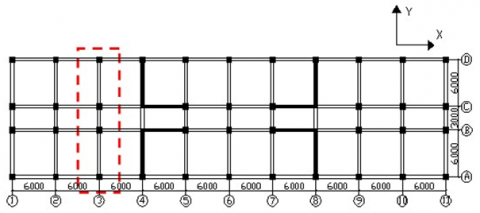Figure 1. Plane layout of the original structure

The concrete strength of shear walls and frame columns is C40 on floors 1-9, and C35 on floors 10-18. The concrete strength of frame beams is C30. On the edges of the frame columns and shear walls, the restraint components adopt HRB400 grade longitudinal steel bars; the steel bars of the frame beams, the longitudinal steel bars of the coupling beams and the steel bars of the shear walls are of HRB335 grade. Moreover, the shear walls are reinforced by 0.3% vertical and horizontal steel bars, and embedded with HPB235 grade stirrups.

## 2.pngFigure 2. Finite-element model of uncontrolled structure

Here, a finite-element model (Figure 2) is established on ABAQUS, in which the wall limbs and coupling beams are simulated as layered shell elements; the frame, wall end columns and concealed columns are simulated as beam elements.

3.2 Seismic response and damage mode

The uncontrolled structure was subject to the seismic load of the El Contro wave with an input peak acceleration of 400gal. The peak inter-floor displacement, and the peak absolute acceleration of each floor are shown in Figure 3 and Figure 4, respectively. The damage indexes of the overall structure and its components are shown in Table 1. The distribution of the damage indexes of the frame-shear wall structure and its components along the floors is shown in Figure 5.

It can be seen that the peak inter-floor displacement of the first floor was much larger than that of any other floor, and the damage indexes of the frame columns and shear walls on the first floor were much greater than those of the beams. Therefore, the structure damage index on the first floor was relatively large, and the overall structure was severely damaged.

In addition, the structural damage mainly concentrated on the 1st, 2nd, 3rd, 6th, and 7th floors, and the wall limbs and frame columns on these floors were damaged more severely than the beams; under large seismic load, the wall limbs, frame columns, and frame beams were damaged slightly, moderately, and slightly, in turn; But the unreasonable damage mode brought severe damages to the overall structure.

## 3.png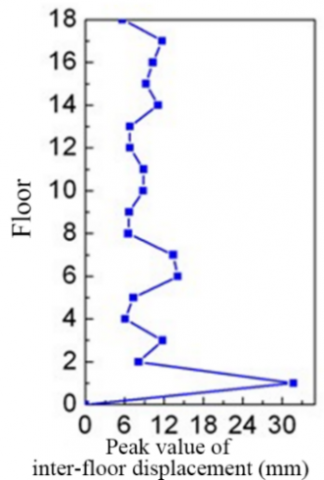Figure 3. Peak inter-floor displacement of each floor

## 4.png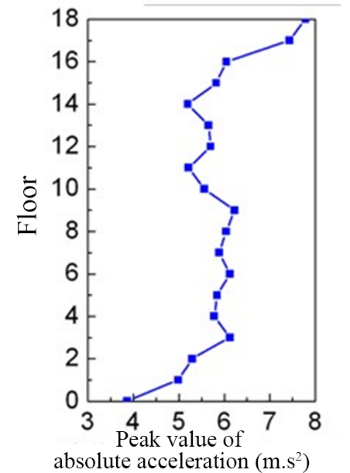Figure 4. Peak acceleration of each floor

Table 1. Damage indexes of the structure and its components

 Wall limbs Frame columns Frame beams Overall structure Damage index 0.274 0.4 0.219 0.784 Damage level Slight Moderate Slight Severe

## 5a.png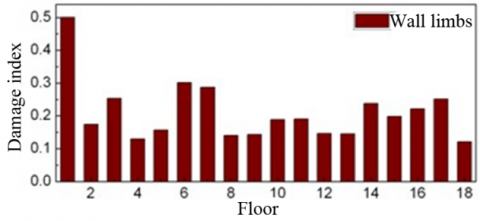(a) Distribution of wall limb damage indexes along the floor

## 5b.png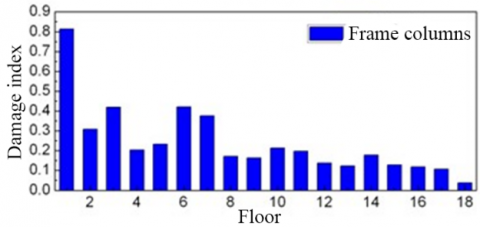(b) Distribution of frame column damage indexes along the floor

## 5c.png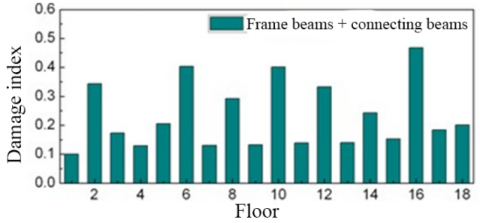(c) Distribution of the sum of frame beam and coupling beam damage indexes along the floor

## 5d.png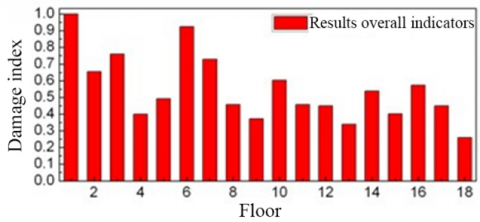(d) Distribution of overall structure damage indexes along the floor

Figure 5. Distribution of damage indexes of overall structure and its components along the floor

4. Damage Mode of Controlled Structure and Parameters of MR Dampers

4.1 Characteristic parameters of MR dampers

The 18-floor frame-shear wall structure with MR dampers was built based on the finite-element model of the uncontrolled structure. The coupling beams were broken in the middle, and MR dampers were added to the broken positions. These dampers were simulated as rod elements. Then, the coupling beams were linked up by lateral rigid connecting rods to transmit axial loads. The length and cross-sectional area of the rod elements were determined according to the preset yield force ratio and yield displacement ratio of the MR dampers. Figure 6 shows the finite-element model of the controlled structure.

## 6.png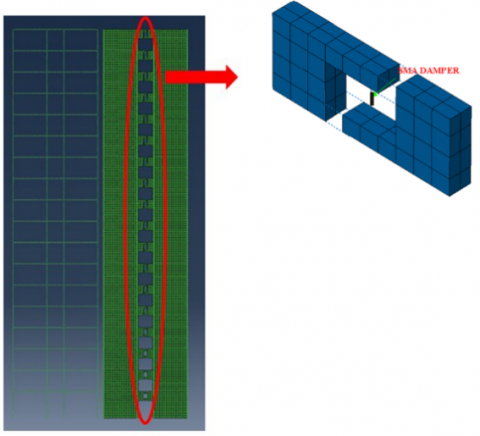Figure 6. Finite-element model of controlled structure

To consume most of the seismic energy, the MR dampers at the middle of the coupling beams should consume energy before the wall limbs yield. This paper takes the yield force ratio of the damper to the coupling beam end (the most vulnerable part of the beam) as a characteristic parameter of the damper. Besides, the yield displacement ratio of the damper to the wall limbs was selected as another characteristic parameter. The two characteristic parameters were calculated as follows:

(1) The yield force ratio of the damper Γ

According to the stress-strain constitutive relationship of the metal rubber materials, the expression of the yield force ratio of the damper is:

$\Gamma =\frac{{{F}_{str}}}{{{F}_{dam}}}=\frac{{{F}_{str}}}{{{\sigma }_{A}}.A}$      (15)

where, Fstr is the maximum shear force of coupling beam end when the structure yields. Its value was obtained by the Pushover method.

(2) The yield displacement ratio of the damper Δ

$\Delta =\frac{{{\Delta }_{str}}}{{{\Delta }_{dam}}}=\frac{{{\Delta }_{str}}}{{{\varepsilon }_{A}}.L}$     (16)

where, Δstr is the relative displacement of coupling beam end obtained through geometrical calculation when the structure yields; Δdam is the damper deformation.

Next, an inverted triangular load was applied on the controlled structure, and pushover analysis was carried out to obtain the static pushover curve. According to the maximum shear force at coupling beam end and the maximum inter-floor displacement when the structure yields, the area A and the length L of the damper were determined. During the parameter analysis, the yield force ratio was set to Γ=0.8, 1.0, and 1.2, in turn; and the yield displacement ratio was set to Δ=0.4, and 0.8 in turn. Hence, a total of six parameter combinations were discussed (Table 2).

Table 2. Parameters of MR dampers in coupling beams of the 18-floor frame-shear structure

 Δ=0.8 Δ=0.4 Γ=0.8 A=0.5475m2, L=33mm A=0.5475 m2, L=55mm Γ=1.0 A=0.438 m2, L=33mm A=0.438 m2, L=55mm Γ=1.2 A=0.365 m2, L=33mm A=0.365 m2, L=55mm

Note: A—damper area, L—damper length

4.2 Influence of damper parameters on the damage of overall structure and its components

Under the Northridge wave with an amplitude of 400 gal, the peak inter-floor displacements of the uncontrolled structure and the controlled structure with different damper parameter combinations are compared in Figure 7. It can be seen that, when damper parameters were Γ=1.2 and Δ=0.4, the response of the structure to large seismic load were controlled well.

Table 3. Damage indexes of overall structure and its components of the uncontrolled and controlled structures

 Wall limbs Frame beams Frame columns Overall structure Uncontrolled structure 0.169 0.149 0.218 0.536 Γ=0.8, Δ=1.0 0.172 0.166 0.221 0.559 Γ=0.8, Δ=0.8 0.158 0.165 0.223 0.546 Γ=1.0, Δ=0.4 0.156 0.147 0.212 0.515 Γ=1.0, Δ=0.8 0.175 0.156 0.229 0.56 Γ=1.2, Δ=0.4 0.142 0.129 0.185 0.456 Γ=1.2, Δ=0.8 0.169 0.151 0.220 0.54

Table 3 compares the damage indexes of overall structure and its components between the uncontrolled structure and the controlled structure with different damper parameter combinations. To reflect the damage control effect of the controlled structure, the damage index change rate R was defined as:

$R=\frac{{{D}_{c}}-{{D}_{N}}}{{{D}_{N}}}$     (17)

where, Dc and DN are the damage indexes of the controlled structure, and the uncontrolled structure, respectively.

## 7a.png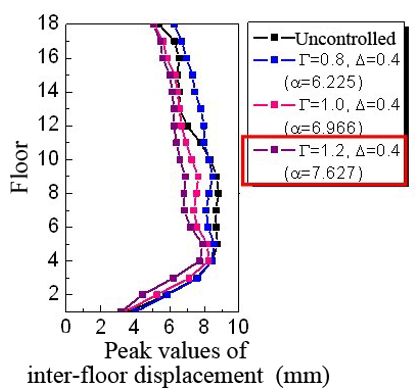(a) Δ=0.4

## 7b.png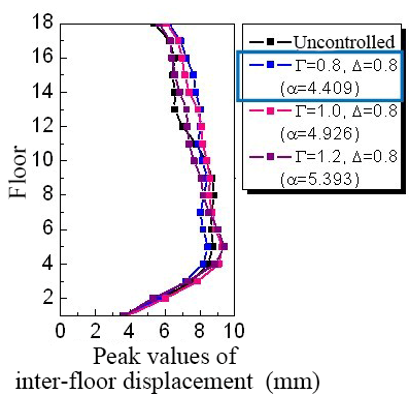(b) Δ=0.8

Figure 7. Peak inter-floor displacements under Northridge wave (PGA=400gal)

## 8a.png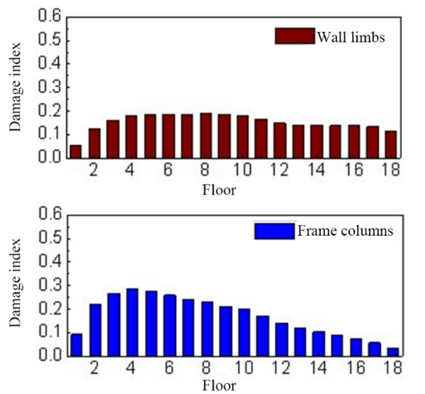## 8b.pngFigure 8. Damage indexes of uncontrolled structure and its components along the floor

## 9a.png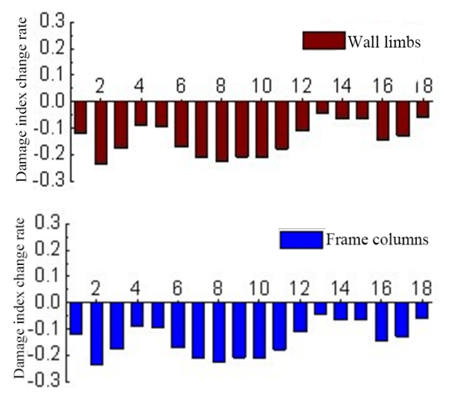## 9b.png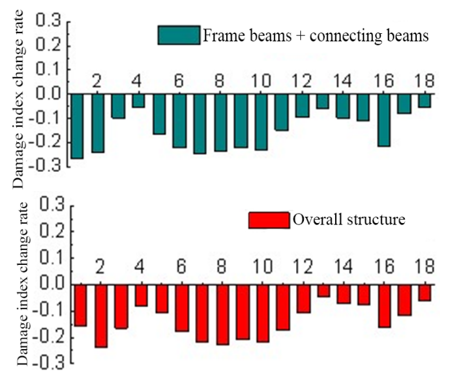Figure 9. Damage index change rates R of a controlled structure and its components along the floor

## 10.png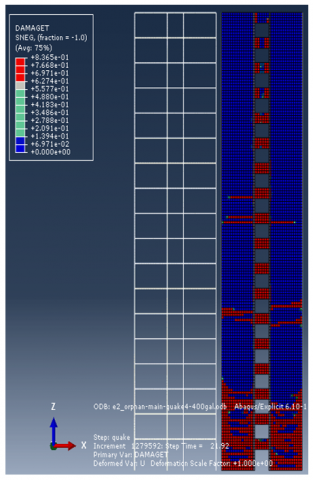Figure 10. Seismic damage cloud of uncontrolled structure

## 11.png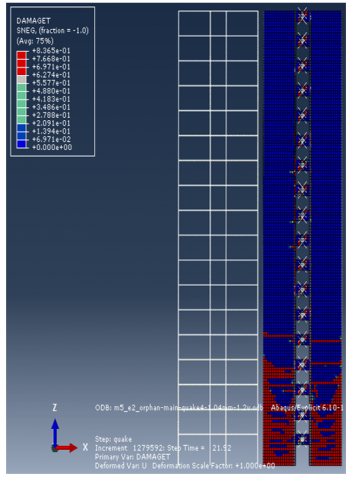Figure 11. Seismic damage cloud of a controlled structure

Figures 8 display the distribution of damage indexes of overall uncontrolled structure and its components along the floor, while Figure 9 present the distribution of R values of the controlled structures with Γ=1.2 and Δ=0.4 and its components along the floor. Figures 10 and 11 are the cloud maps of the concrete tensile damage of uncontrolled and controlled structures, respectively.

As shown in Figure 9, the change rates of damage indexes of controlled structure were all negative, suggesting that the damage of the controlled structure with installed dampers was controlled to a certain extent. When damper parameters were Γ=1.2 and Δ=0.4, the inter-floor displacement, as well as the damages of local components and the overall structure were controlled effectively.

Comparing the damage indexes of overall structure and its components, as long as the parameters were in a reasonable range, the control effect of the dampers improved with the growing damper stiffness.

As shown in Figure 10, the structural damage mainly concentrated on the 2nd, 3rd, 6th, and 10th floors. The wall limbs and frame columns of these floors were damaged more severely than the beams.

From Figures 10 and 11, it is learned that the damper installation improved the control of structure damage.

5. Conclusions

Drawing on Park and Ang’s damage model, this paper presents formulas of damage indexes based on the actual experimental data on different components, and then calculated the damage indexes of the overall structure and its component. After that, an 18-floor frame-shear wall structure was modeled on ABAQUS with and without dampers. The parameters of uncontrolled and controlled structures were compared in details. The main conclusions are as follows:

(1) The component damage indexes were derived from the ultimate damage curvature, peak deformation, and cumulative hysteretic energy consumption.

(2) The correspondence between damage level of target structure, reasonable damage mode, and damage index of uncontrolled and controlled frame-shear wall structures were obtained under seismic waves of different intensities.

(3) A total of six combinations of damper parameters were designed for parameter analysis: yield force ratio=0.8, 1.0, 1.2, and yield displacement ratio =0.4, 0.8. Under the Northridge wave with a peak acceleration of 400 gal, the peak inter-floor displacements of uncontrolled structures with different parameter combinations were compared, revealing that the structure response to large seismic load could be controlled well at the damper parameters Γ=1.2 and Δ=0.4.

(4) The damage indexes of uncontrolled structure and its components, as well as the damage index change rate of a controlled structure along the floor were examined carefully. The results show that the optimal damage control effect on the structure and its components was achieved at the damper parameters Γ=1.2 and Δ=0.4.

Acknowledgment

This work is supported by Heilongjiang Provincial Natural Science Foundation for the general project “Seismic Failure Modes of frame-shear Structures in Energy Dissipators Based on Metal Pseudo-Rubber / Silicone Rubber” (Grant No.: E201401).

References

 Fu, C., Li, Y., Sun, X., Xu, J. (2010). Experimental study on seismic performance of prestressed and non-prestressed steel reinforced concrete frames. Journal of Building Structures, 31(8): 15-21.

 Heredia-Zavoni, E., Zeballos, A., Esteva, L. (2000). Theoretical models and recorded response in the estimation of cumulative seismic damage on non-linear structures. Earthquake Engineering & Structural Dynamics, 29(12): 1779-1796. https://doi.org/10.1002/1096-9845(200012)29:12%3C1779::AID-EQE985%3E3.0.CO;2-F

 Alfarah, B., López-Almansa, F., Oller, S. (2017). New methodology for calculating damage variables evolution in Plastic Damage Model for RC structures. Engineering Structures, 132: 70-86. https://doi.org/10.1016/j.engstruct.2016.11.022

 Li, J., Liang, L., Liu, X., Ma, H., Song, J., Wei, Y. (2018). Experimental studies on strengthening and failure mechanism for the metal/silicone rubber/metal bonding system. International Journal of Applied Mechanics, 10(3): 1850029. https://doi.org/10.1142/S1758825118500291

 Ponomarev, Y.K., Ermakov, A.I., Simakov, O.B., Mikhalkin, I.K. (2013). Metallic counterpart of rubber: A material for vibration and shock protection. Metal Science and Heat Treatment, 55(1-2): 8-13. https://doi.org/10.1007/s11041-013-9570-3

 Ren, Z.Y., Chen, Q., Bai, H., Wu, Y. (2018). Study on damping energy dissipation characteristics of cylindrical metal rubber in nonforming direction. Advances in Materials Science and Engineering, 2018: 5014789. https://doi.org/10.1155/2018/5014789

 Xiao, S., Xu, L., Lu, X. (2018). Nonlinear damage model for seismic damage assessment of reinforced concrete frame members and structures. Acta Mechanica Sinica, 34(5): 949-962. https://doi.org/10.1007/s10409-018-0779-7

 Zhang, P., Hou, S., Ou, J. (2016). A beam–column joint element for analysis of reinforced concrete frame structures. Engineering Structures, 118: 125-136. https://doi.org/10.1016/j.engstruct.2016.03.030

 Shokrabadi, M., Banazadeh, M., Shokrabadi, M., Mellati, A. (2015). Assessment of seismic risks in code conforming reinforced concrete frames. Engineering Structures, 98: 14-28. https://doi.org/10.1016/j.engstruct.2015.03.057

 Bertero, R.D., Bertero, V.V. (2002). Performance-based seismic engineering: the need for a reliable conceptual comprehensive approach. Earthquake Engineering & Structural Dynamics, 31(3): 627-652. https://doi.org/10.1002/eqe.146

 Burlion, N., Gatuingt, F., Pijaudier-Cabot, G., Daudeville, L. (2000). Compaction and tensile damage in concrete: constitutive modelling and application to dynamics. Computer Methods in Applied Mechanics and Engineering, 183(3-4): 291-308. https://doi.org/10.1016/S0045-7825(99)00223-6

 Carol, I., Rizzi, E., Willam, K. (2001). On the formulation of anisotropic elastic degradation.: II. Generalized pseudo-Rankine model for tensile damage. International Journal of Solids and Structures, 38(4): 519-546. https://doi.org/10.1016/S0020-7683(00)00031-7

 Faria, R., Oliver, J., Cervera, M. (1998). A strain-based plastic viscous-damage model for massive concrete structures. International Journal of Solids and Structures, 35(14): 1533-1558. https://doi.org/10.1016/S0020-7683(97)00119-4

 Häussler-Combe, U., Hartig, J. (2008). Formulation and numerical implementation of a constitutive law for concrete with strain-based damage and plasticity. International Journal of Non-Linear Mechanics, 43(5): 399-415. https://doi.org/10.1016/j.ijnonlinmec.2008.01.005

 Jason, L., Huerta, A., Pijaudier-Cabot, G., Ghavamian, S. (2006). An elastic plastic damage formulation for concrete: Application to elementary tests and comparison with an isotropic damage model. Computer Methods in Applied Mechanics and Engineering, 195(52): 7077-7092. https://doi.org/10.1016/j.cma.2005.04.017

 Krätzig, W.B., Pölling, R. (2004). An elasto-plastic damage model for reinforced concrete with minimum number of material parameters. Computers & Structures, 82(15-16): 1201-1215. https://doi.org/10.1016/j.compstruc.2004.03.002

 Nguyen, G.D., Korsunsky, A.M. (2008). Development of an approach to constitutive modelling of concrete: isotropic damage coupled with plasticity. International Journal of Solids and Structures, 45(20): 5483-5501. https://doi.org/10.1016/j.ijsolstr.2008.05.029

 Li, G., Zhang, Y., Li, H.N. (2013). Seismic damage analysis of reinforced concrete frame using the force analogy method. Journal of Engineering Mechanics, 139(12): 1780-1789. https://doi.org/10.1061/(ASCE)EM.1943-7889.0000618

 Ibarra, L.F., Medina, R.A., Krawinkler, H. (2005). Hysteretic models that incorporate strength and stiffness deterioration. Earthquake Engineering & Structural Dynamics, 34(12): 1489-1511. https://doi.org/10.1002/eqe.495

 Ou, J.P., Niu, D.T., Wang, G.Y. (1999). Loss assessment and optimum design of multi-storey nonlinear seismic structures. Computational Structural Mechanics and Application, 9(2): 171-179.

 Park, Y.J., Ang, A.H.S. (1985). Mechanistic seismic damage model for reinforced concrete. Journal of Structural Engineering, 111(4): 722-739. https://doi.org/10.1061/(ASCE)0733-9445(1985)111:4(722)